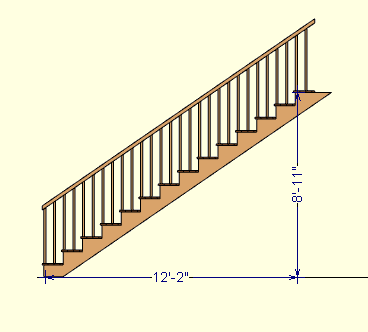Spike's Calculators

# Slope in Degrees from Rise and Run

Calculate the angle from a rise and run measurement. Can be used for anything that can be measured with a horizontal and vertical length measurement, like driveways, roofs, stairways, gable walls, etc..

##### Example:

This calculator uses the rise and run with the arctan function to calculate the slope in degrees.

A stairway has a run of 12' - 2" and a total rise of 8' - 11"
convert these amounts to inches
12' - 2" = 146 inches of run
8' - 11" = 107 inches of rise
divide the rise by the run
107 ÷ 146 = 0.7328767......
plug 0.7328767....... into an arctan calculation and the result = 36.2369
The stairway is built at an angle of 36.2°### Rise and Run/Arctan Function

Run ft in
Rise ft in
Decimal Precision #